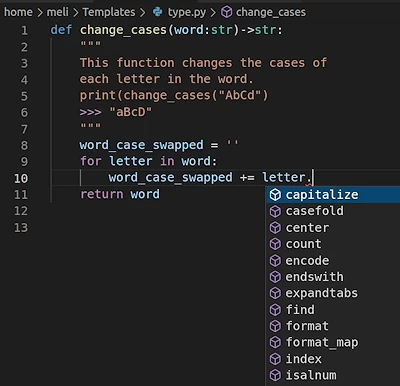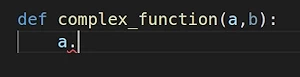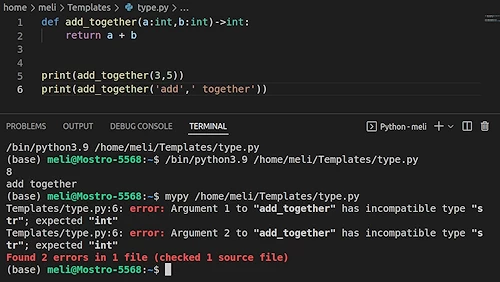# 7. Type Annotations

If you know another programming language such as C or C++, you are used to declaring what data type you are working with. For example, this is how you would declare an integer in C.

int a;


from this moment on, we know that "a" is of type integer. Then we would maybe assign an integer to a.

 a = 3


However we don't do it like that in Python. Let's go through what we already know.

a = 3
print(type(a))


### OUTPUT:

<class 'int'>


As a is assigned an integer, it belongs to the integer class itself, without us having to say

 a = int()


beforehand. a's data type would change accordingly, when it is assigned values from other data types.

a = 'hello'
print(type(a))


### OUTPUT:

<class 'str'>

a = 3.14
print(type(a))


### OUTPUT:

<class 'float'>


If Python is capable of determining the types itself, why are even type annotations useful?

## Type Annotations: Why

• You understand where the type errors stem from.
• If you are using an IDE, such as Visual Studio Code, typing the type annotations would allow you to access the built-in functions easier.• When a variable is of no type, you wouldn't be able to automatically access the built-in functions that you intend to use.• You would get syntax-highlighting as a warning before you even run your code.

• Your code will be more readable & understandable. This is especially useful if you are working together with others.

Live Python training

Enjoying this page? We offer live Python training courses covering the content of this site.

Enrol here

## Type Annotations: How

### Example 1

In this example you see the ord()function, which takes a string as input and converts it into an integer by using the ASCII values of the letters it contains.

def my_function(a:int,b:str)->int:
return a + ord(b)

print(my_function(3,'a'))


### OUTPUT:

100


my_function() works perfectly, since b has to be of type string and it is of type string. Let's try it otherwise:

print(my_function(3,5))


### OUTPUT:

---------------------------------------------------------------------------
TypeError                                 Traceback (most recent call last)
/tmp/ipykernel_19492/488431691.py in <module>
----> 1 print(my_function(3,5))

/tmp/ipykernel_19492/2046822703.py in my_function(a, b)
1 def my_function(a:int,b:str)->int:
----> 2     return a + ord(b)

TypeError: ord() expected string of length 1, but int found


Here we receive a TypeError because we wrote an integer, and not a string for b. Since ord() needs a string to function, the code doesn't work.

print(my_function('a','a'))


### OUTPUT:

---------------------------------------------------------------------------
TypeError                                 Traceback (most recent call last)
/tmp/ipykernel_19492/1936946746.py in <module>
----> 1 print(my_function('a','a'))

/tmp/ipykernel_19492/2046822703.py in my_function(a, b)
1 def my_function(a:int,b:str)->int:
----> 2     return a + ord(b)

TypeError: can only concatenate str (not "int") to str


Here we receive another TypeError because we wrote a string, and not an integer for a. While b is sucessfully converted into an integer by the ord() function, it is yet not possible to concatenate strings and integers.

print(my_function(4.2,'a'))


### OUTPUT:

101.2


Interestingly our function runs now, yet our argument a is of type float and not integer as we declared at the very beginning.

### Example 2:

In this example, we are going to see how the functions can be given variables that can work without raising any errors. However, that can be still be very problematic.

def add_together(a:int,b:int)->int:
return a + b

def return_the_last_digit(a:int)->int:
return a % 10

our_sum = add_together(38,57)
print(return_the_last_digit(our_sum))


### OUTPUT:

5

our_sum = add_together('add',' together')

print(return_the_last_digit(our_sum))


### OUTPUT:

---------------------------------------------------------------------------
TypeError                                 Traceback (most recent call last)
/tmp/ipykernel_19492/576833914.py in <module>
----> 1 print(return_the_last_digit(our_sum))

/tmp/ipykernel_19492/1809188113.py in return_the_last_digit(a)
1 def return_the_last_digit(a:int)->int:
----> 2     return a % 10

TypeError: not all arguments converted during string formatting


## How can we run a TypeError check before we actually run the program?

By installing mypy and running it before you run your code, you could avoid type errors. Mypy checks your annotations and gives a warning if a function is initialized with the wrong datatype.All in all, type annotations are very useful and it can save a lot of time for you and it can make your code readable for both yourself and the others.

Live Python training

Enjoying this page? We offer live Python training courses covering the content of this site.

Upcoming online Courses

Enrol here